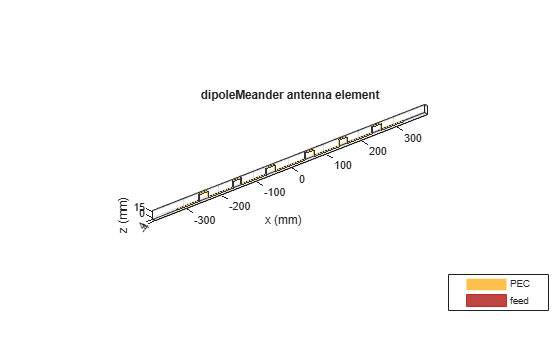# dipoleMeander

Create meander dipole antenna

## Description

The `dipoleMeander` class creates a meander dipole antenna with four dipoles. The antenna is center fed and it is symmetric about its origin. The first resonance of meander dipole antenna is at 200 MHz.

The width of the dipole is related to the diameter of an equivalent cylindrical dipole by the equation

`$w=2d=4r$`

, where:

• d is the diameter of equivalent cylindrical dipole.

• r is the radius of equivalent cylindrical dipole.

For a given cylinder radius, use the `cylinder2strip` utility function to calculate the equivalent width. The default strip dipole is center-fed. The feed point coincides with the origin. The origin is located on the xy- plane.## Creation

### Syntax

``dm = dipoleMeander``
``dm = dipoleMeander(Name,Value)``

### Description

example

``` `dm = dipoleMeander` creates a meander dipole antenna with four dipoles.```
``` `dm = dipoleMeander(Name,Value)` creates a meander dipole antenna with four dipoles, with additional properties specified by one or more name-value pair arguments. `Name` is the property name and `Value` is the corresponding value. You can specify several name-value pair arguments in any order as `Name1`, `Value1`, `...`, `NameN`, `ValueN`. Properties not specified retain their default values.```

## Properties

expand all

Dipole width, specified as a scalar in meters.

Example: `'Width',0.05`

Data Types: `double`

Length of individual dipole arms, specified as a vector in meters. The total number of dipole arms generated is :

`$2\ast N-1$`

where N is the number of specified arm lengths.

Example: ```'ArmLength',[0.6000 0.5000 1 0.4000]```

Data Types: `double`

Notch length along the length of the antenna, specified as a scalar in meters.

For example, in a dipole meander antenna with seven stacked arms there are six notches.

Example: `'NotchLength',1`

Data Types: `double`

Notch width perpendicular to the length of the antenna, specified as a scalar in meters.

Example: `'NotchWidth',1`

Data Types: `double`

Type of the metal used as a conductor, specified as a metal material object. You can choose any metal from the `MetalCatalog` or specify a metal of your choice. For more information, see `metal`. For more information on metal conductor meshing, see Meshing.

Example: ```m = metal('Copper'); 'Conductor',m```

Example: ```m = metal('Copper'); ant.Conductor = m```

Lumped elements added to the antenna feed, specified as a lumped element object. For more information, see `lumpedElement`.

Example: `'Load',lumpedelement`. `lumpedelement` is the object for the load created using `lumpedElement`.

Example: ```dm.Load = lumpedElement('Impedance',75)```

Tilt angle of the antenna in degrees, specified as a scalar or vector. For more information, see Rotate Antennas and Arrays.

Example: `90`

Example: `Tilt=[90 90]`,`TiltAxis=[0 1 0;0 1 1]` tilts the antenna at 90 degrees about the two axes defined by the vectors.

Data Types: `double`

Tilt axis of the antenna, specified as one of these values:

• Three-element vector of Cartesian coordinates in meters. In this case, each coordinate in the vector starts at the origin and lies along the specified points on the x-, y-, and z-axes.

• Two points in space, specified as a 2-by-3 matrix corresponding to two three-element vectors of Cartesian coordinates. In this case, the antenna rotates around the line joining the two points.

• `"x"`, `"y"`, or `"z"` to describe a rotation about the x-, y-, or z-axis, respectively.

Example: `[0 1 0]`

Example: `[0 0 0;0 1 0]`

Example: `"Z"`

Data Types: `double` | `string`

## Object Functions

 `show` Display antenna, array structures, shapes, or platform `info` Display information about antenna or array `axialRatio` Axial ratio of antenna `beamwidth` Beamwidth of antenna `charge` Charge distribution on antenna or array surface `current` Current distribution on antenna or array surface `design` Design prototype antenna or arrays for resonance around specified frequency or create AI-based antenna from antenna catalog objects `efficiency` Radiation efficiency of antenna `EHfields` Electric and magnetic fields of antennas or embedded electric and magnetic fields of antenna element in arrays `impedance` Input impedance of antenna or scan impedance of array `mesh` Mesh properties of metal, dielectric antenna, or array structure `meshconfig` Change meshing mode of antenna, array, custom antenna, custom array, or custom geometry `optimize` Optimize antenna or array using SADEA optimizer `pattern` Plot radiation pattern and phase of antenna or array or embedded pattern of antenna element in array `patternAzimuth` Azimuth plane radiation pattern of antenna or array `patternElevation` Elevation plane radiation pattern of antenna or array `rcs` Calculate and plot radar cross section (RCS) of platform, antenna, or array `returnLoss` Return loss of antenna or scan return loss of array `sparameters` Calculate S-parameters for antennas and antenna arrays `vswr` Voltage standing wave ratio (VSWR) of antenna or array element

## Examples

collapse all

Create and view the default meander dipole antenna.

`dm = dipoleMeander`
```dm = dipoleMeander with properties: Width: 0.0040 ArmLength: [0.0880 0.0710 0.0730 0.0650] NotchLength: 0.0238 NotchWidth: 0.0170 Conductor: [1x1 metal] Tilt: 0 TiltAxis: [1 0 0] Load: [1x1 lumpedElement] ```
`show(dm)`Plot the radiation pattern of meander dipole antenna at a 200 MHz frequency.

```dm = dipoleMeander; pattern(dm,200e6)```Balanis, C.A. Antenna Theory: Analysis and Design. 3rd Ed. New York: Wiley, 2005.